## CSMA / CD Protocol-

Before you go through this article, make sure that you have gone through the previous article on CSMA / CD Protocol.

We have discussed-

• CSMA / CD allows a station to transmit data if it senses the carrier free.
• After undergoing collision, station waits for random back off time before transmitting again.
• Back Off Algorithm is used to calculate back off time.

In this article, we will discuss practice problems based on CSMA / CD and Back Off Algorithm.

## Problem-01:

After the kth consecutive collision, each colliding station waits for a random time chosen from the interval-

1. (0 to 2k) x RTT
2. (0 to 2k-1) x RTT
3. (0 to 2k-1) x Maximum Propagation delay
4. (0 to 2k-1) x Maximum Propagation delay

## Solution-

Clearly, Option (B) is correct.

## Problem-02:

In a CSMA / CD network running at 1 Gbps over 1 km cable with no repeaters, the signal speed in the cable is 200000 km/sec. What is minimum frame size?

## Solution-

Given-

• Bandwidth = 1 Gbps
• Distance = 1 km
• Speed = 200000 km/sec

### Calculating Propagation Delay-

Propagation delay (Tp)

= Distance / Propagation speed

= 1 km / (200000 km/sec)

= 0.5 x 10-5 sec

= 5 x 10-6 sec

### Calculating Minimum Frame Size-

Minimum frame size

= 2 x Propagation delay x Bandwidth

= 2 x 5 x 10-6 sec x 109 bits per sec

= 10000 bits

## Problem-03:

A 2 km long broadcast LAN has 107 bps bandwidth and uses CSMA / CD. The signal travels along the wire at 2 x 108 m/sec. What is the minimum packet size that can be used on this network?

1. 50 B
2. 100 B
3. 200 B
4. None of the above

## Solution-

Given-

• Distance = 2 km
• Bandwidth = 107 bps
• Speed = 2 x 108 m/sec

### Calculating Propagation Delay-

Propagation delay (Tp)

= Distance / Propagation speed

= 2 km / (2 x 108 m/sec)

= 2 x 103 m / (2 x 108 m/sec)

= 10-5 sec

### Calculating Minimum Frame Size-

Minimum frame size

= 2 x Propagation delay x Bandwidth

= 2 x 10-5 sec x 107 bits per sec

= 200 bits or 25 bytes

Thus, Option (D) is correct.

## Problem-04:

A and B are the only two stations on Ethernet. Each has a steady queue of frames to send. Both A and B attempts to transmit a frame, collide and A wins first back off race. At the end of this successful transmission by A, both A and B attempt to transmit and collide. The probability that A wins the second back off race is ___ .

1. 0.5
2. 0.625
3. 0.75
4. 1.0

## Solution-

According to question, we have-

### 1st Transmission Attempt-

• Both the stations A and B attempts to transmit a frame.
• A collision occurs.
• Back Off Algorithm runs.
• Station A wins and successfully transmits its 1st data packet.

### 2nd Transmission Attempt-

• Station A attempts to transmit its 2nd data packet.
• Station B attempts to retransmit its 1st data packet.
• A collision occurs.

Now,

• We have been asked the probability of station A to transmit its 2nd data packet successfully after 2nd collision.
• After the 2nd collision occurs, we have-

### At Station A-

• 2nd data packet of station A undergoes collision for the 1st time.
• So, collision number for the 2nd data packet of station A = 1.
• Now, station A randomly chooses a number from the range [0,21-1] = [0,1].
• Then, station A waits for back off time and then attempts to retransmit its data packet.

### At Station B-

• 1st data packet of station B undergoes collision for the 2nd time.
• So, collision number for the 1st data packet of station B = 2.
• Now, station B randomly chooses a number from the range [0,22-1] = [0,3].
• Then, station B waits for back off time and then attempts to retransmit its data packet.

Following 8 cases are possible-

 Station A Station B Remark 0 0 Collision 0 1 A wins 0 2 A wins 0 3 A wins 1 0 B wins 1 1 Collision 1 2 A wins 1 3 A wins

From here,

• Probability of A winning the 2nd back off race = 5 / 8 = 0.625.
• Thus, Option (B) is correct.

## Problem-05:

Suppose nodes A and B are on same 10 Mbps Ethernet segment and the propagation delay between two nodes is 225 bit times. Suppose A and B send frames at t=0, the frames collide then at what time, they finish transmitting a jam signal. Assume a 48 bit jam signal.

## Solution-

Propagation delay (Tp)

= 225 bit times

= 225 bit / 10 Mbps

= 22.5 x 10-6 sec

= 22.5 μsec

### At t = 0,

• Nodes A and B start transmitting their frame.
• Since both the stations start simultaneously, so collision occurs at the mid way.
• Time after which collision occurs = Half of propagation delay.
• So, time after which collision occurs = 22.5 μsec / 2 = 11.25 μsec.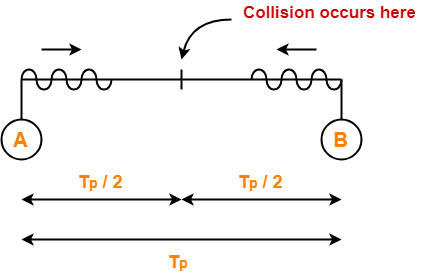### At t = 11.25 μsec,

• After collision occurs at t = 11.25 μsec, collided signals start travelling back.
• Collided signals reach the respective nodes after time = Half of propagation delay
• Collided signals reach the respective nodes after time = 22.5 μsec / 2 = 11.25 μsec.
• Thus, at t = 22.5 μsec, collided signals reach the respective nodes.

### At t = 22.5 μsec,

• As soon as nodes discover the collision, they immediately release the jam signal.
• Time taken to finish transmitting the jam signal = 48 bit time = 48 bits/ 10 Mbps = 4.8 μsec.

Thus,

Time at which the jam signal is completely transmitted

= 22.5 μsec + 4.8 μsec

= 27.3 μsec or 273 bit times

## Problem-06:

Suppose nodes A and B are attached to opposite ends of the cable with propagation delay of 12.5 ms. Both nodes attempt to transmit at t=0. Frames collide and after first collision, A draws k=0 and B draws k=1 in the exponential back off protocol. Ignore the jam signal. At what time (in seconds), is A’s packet completely delivered at B if bandwidth of the link is 10 Mbps and packet size is 1000 bits.

## Solution-

Given-

• Propagation delay = 12.5 ms
• Bandwidth = 10 Mbps
• Packet size = 1000 bits

### Time At Which Collision Occurs-

Collision occurs at the mid way after time

= Half of Propagation delay

= 12.5 ms / 2

= 6.25 ms

Thus, collision occurs at time t = 6.25 ms.

### Time At Which Collision is Discovered-

Collision is discovered in the time it takes the collided signals to reach the nodes

= Half of Propagation delay

= 12.5 ms / 2

= 6.25 ms

Thus, collision is discovered at time t = 6.25 ms + 6.25 ms = 12.5 ms.

### Scene After Collision-

After the collision is discovered,

• Both the nodes wait for some random back off time.
• A chooses k=0 and then waits for back off time = 0 x 25 ms = 0 ms.
• B chooses k=1 and then waits for back off time = 1 x 25 ms = 25 ms.
• From here, A begins retransmission immediately while B waits for 25 ms.

### Waiting Time For A-

• After winning the back off race, node A gets the authority to retransmit immediately.
• But node A does not retransmit immediately.
• It waits for the channel to clear from the last bit aborted by it on discovering the collision.
• Time taken by the last bit to get off the channel = Propagation delay = 12.5 ms.
• So, node A waits for time = 12.5 ms and then starts the retransmission.
• Thus, node A starts the retransmission at time t = 12.5 ms + 12.5 ms = 25 ms.

### Time Taken in Delivering Packet To Node B-

Time taken to deliver the packet to node B

= Transmission delay + Propagation delay

= (1000 bits / 10 Mbps) + 12.5 ms

= 100 μs + 12.5 ms

= 0.1 ms + 12.5 ms

= 12.6 ms

Thus, At time t = 25 ms + 12.6 ms = 37.6 ms, the packet is delivered to node B.

## Problem-07:

The network consists of 4 hosts distributed as shown below-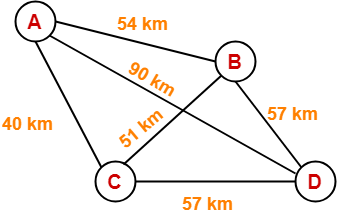Assume this network uses CSMA / CD and signal travels with a speed of 3 x 105 km/sec. If sender sends at 1 Mbps, what could be the minimum size of the packet?

1. 600 bits
2. 400 bits
3. 6000 bits
4. 1500 bits

## Solution-

• CSMA / CD is a Access Control Method.
• In the given network, all the links are point to point.
• So, there is actually no need of implementing CSMA / CD.
• Stations can transmit whenever they want to transmit.

 In CSMA / CD,The condition to detect collision is-Packet size >= 2 x (distance / speed) x Bandwidth

To solve the question,

• We assume that a packet of same length has to be used in the entire network.
• To get the minimum length of the packet, what distance we should choose?
• To get the minimum length of the packet, we should choose the minimum distance.
• But, then collision would be detected only in the links having distance less than or equal to that minimum distance.
• For the links, having distance greater than the minimum distance, collision would not be detected.
• So, we choose the maximum distance so that collision can be detected in all the links of the network.

So, we use the values-

• Distance = 90 km
• Speed = 3 x 105 km/sec
• Bandwidth = 1 Mbps

Substituting these values, we get-

Minimum size of data packet

= 2 x (90 km / 3 x 105 km per sec) x 1 Mbps

= 2 x 30 x 10-5 sec x 106 bits per sec

= 600 bits

Thus, Option (A) is correct.

Next Article- Token Passing | Access Control Method

Get more notes and other study material of Computer Networks.

Watch video lectures by visiting our YouTube channel LearnVidFun.

## Access Control in Networking-

Before you go through this article, make sure that you have gone through the previous article on Access Control.

We have discussed-

• Access Control is a mechanism that controls the access of stations to the transmission link.
• There are various access control methods-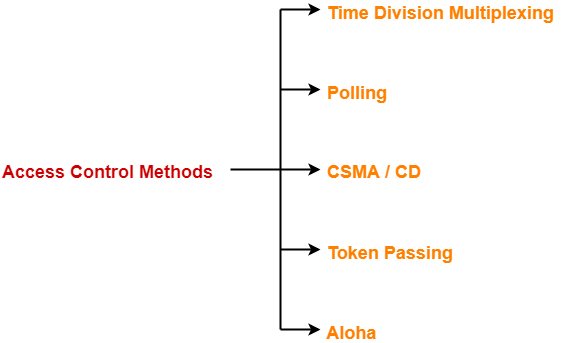## Aloha-

There are two different versions of Aloha-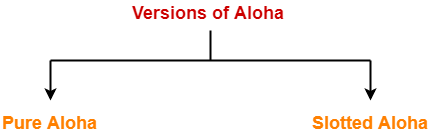1. Pure Aloha
2. Slotted Aloha

## 1. Pure Aloha-

• It allows the stations to transmit data at any time whenever they want.
• After transmitting the data packet, station waits for some time.

Then, following 2 cases are possible-

## Case-01:

• Transmitting station receives an acknowledgement from the receiving station.
• In this case, transmitting station assumes that the transmission is successful.

## Case-02:

• Transmitting station does not receive any acknowledgement within specified time from the receiving station.
• In this case, transmitting station assumes that the transmission is unsuccessful.

Then,

• Transmitting station uses a Back Off Strategy and waits for some random amount of time.
• After back off time, it transmits the data packet again.
• It keeps trying until the back off limit is reached after which it aborts the transmission.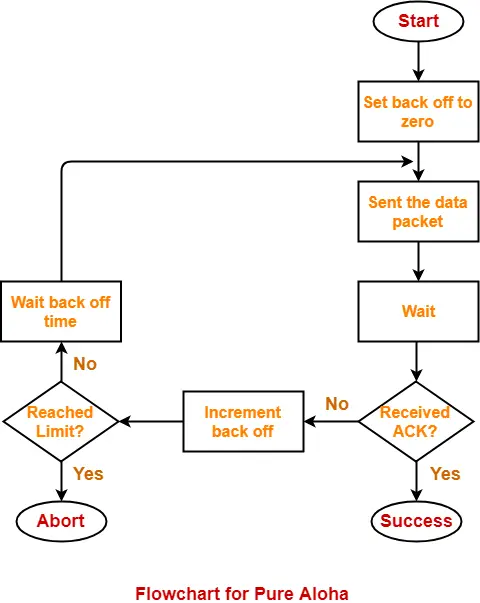## Efficiency-

 Efficiency of Pure Aloha (η) = G x e-2G

where G = Number of stations willing to transmit data

## Maximum Efficiency-

For maximum efficiency,

• We put dη / dG = 0
• Maximum value of η occurs at G = 1/2
• Substituting G = 1/2 in the above expression, we get-

Maximum efficiency of Pure Aloha

= 1/2 x e-2 x 1/2

= 1 / 2e

= 0.184

= 18.4%

Thus,

 Maximum Efficiency of Pure Aloha (η) = 18.4%

The maximum efficiency of Pure Aloha is very less due to large number of collisions.

## 2. Slotted Aloha-

• Slotted Aloha divides the time of shared channel into discrete intervals called as time slots.
• Any station can transmit its data in any time slot.
• The only condition is that station must start its transmission from the beginning of the time slot.
• If the beginning of the slot is missed, then station has to wait until the beginning of the next time slot.
• A collision may occur if two or more stations try to transmit data at the beginning of the same time slot.

## Efficiency-

 Efficiency of Slotted Aloha (η) = G x e-G

where G = Number of stations willing to transmit data at the beginning of the same time slot

### Maximum Efficiency-

For maximum efficiency,

• We put dη / dG = 0
• Maximum value of η occurs at G = 1
• Substituting G = 1 in the above expression, we get-

Maximum efficiency of Slotted Aloha

= 1 x e-1

= 1 / e

= 0.368

= 36.8%

Thus,

 Maximum Efficiency of Slotted Aloha (η) = 36.8%

The maximum efficiency of Slotted Aloha is high due to less number of collisions.

## Difference Between Pure Aloha And Slotted Aloha-

 Pure Aloha Slotted Aloha Any station can transmit the data at any time. Any station can transmit the data at the beginning of any time slot. The time is continuous and not globally synchronized. The time is discrete and globally synchronized. Vulnerable time in which collision may occur= 2 x Tt Vulnerable time in which collision may occur= Tt Probability of successful transmission of data packet= G x e-2G Probability of successful transmission of data packet= G x e-G Maximum efficiency = 18.4%(Occurs at G = 1/2) Maximum efficiency = 36.8%( Occurs at G = 1) The main advantage of pure aloha is its simplicity in implementation. The main advantage of slotted aloha is that it reduces the number of collisions to half and doubles the efficiency of pure aloha.

## Problem-

A group of N stations share 100 Kbps slotted ALOHA channel. Each station output a 500 bits frame on an average of 5000 ms even if previous one has not been sent. What is the required value of N?

## Solution-

### Throughput Of One Station-

Throughput of each station

= Number of bits sent per second

= 500 bits / 5000 ms

= 500 bits / (5000 x 10-3 sec)

= 100 bits/sec

### Throughput Of Slotted Aloha-

Throughput of slotted aloha

= Efficiency x Bandwidth

= 0.368 x 100 Kbps

= 36.8 Kbps

### Total Number Of Stations-

Throughput of slotted aloha = Total number of stations x Throughput of each station

Substituting the values, we get-

36.8 Kbps = N x 100 bits/sec

∴ N = 368

Thus, required value of N = 368.

To gain better understanding about Aloha,

Watch this Video Lecture

Next Article- Ethernet | Ethernet Frame Format

Get more notes and other study material of Computer Networks.

Watch video lectures by visiting our YouTube channel LearnVidFun.

## Access Control in Networking-

Before you go through this article, make sure that you have gone through the previous article on Access Control.

We have discussed-

• Access Control is a mechanism that controls the access of stations to the transmission link.
• There are various access control methods-Before discussing Token Passing, let us discuss few important concepts required for the discussion.

## Time Conversions-

In token passing,

• Time may be expressed in seconds, bits or meters.
• To convert the time from one unit to another, we use the following conversion chart-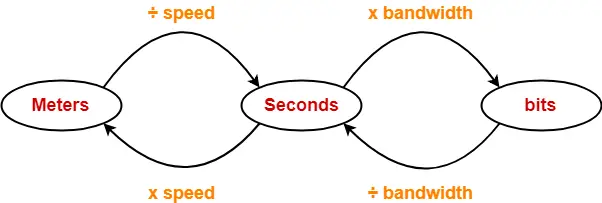## Token Passing Terminology-

The following terms are frequently used-

1. Token
2. Ring Latency
3. Cycle Time

## 1. Token-

• A token is a small message composed of a special bit pattern.
• It represents the permission to send the data packet.
• A station is allowed to transmit a data packet if and only if it possess the token otherwise not.

## 2. Ring Latency-

 Time taken by a bit to complete one revolution of the ring is called as ring latency.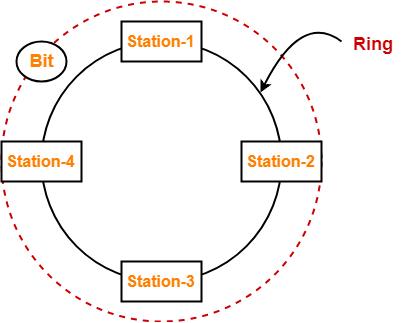Let us derive the expression for ring latency.

If-

• Length of the ring = d
• Speed of the bit = v
• Number of stations = N
• Bit delay at each station = b

(Bit delay is the time for which a station holds the bit before transmitting to the other side)

Then-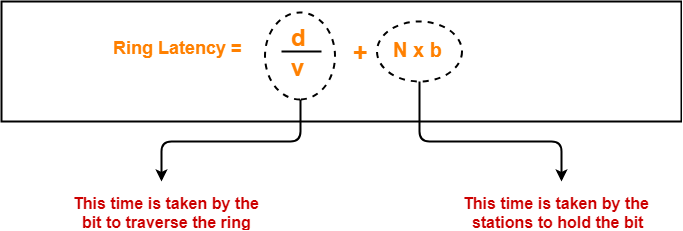## Notes-

• d / v is the propagation delay (Tp) expressed in seconds.
• Generally, bit delay is expressed in bits.
• So, both the terms (d / v and N x b) have different units.
• While calculating the ring latency, both the terms are brought into the same unit.
• The above conversion chart is used for conversion.

After conversion, we have-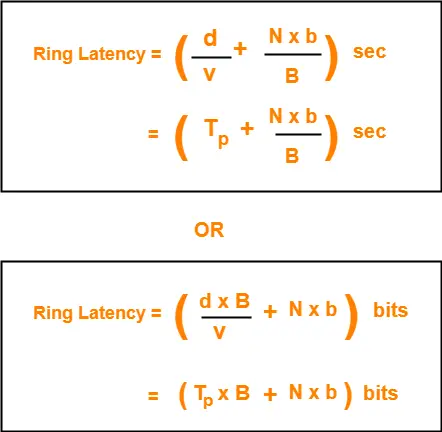## 3. Cycle Time-

 Time taken by the token to complete one revolution of the ring is called as cycle time.

If-

• Length of the ring = d
• Speed of the bit = v
• Number of stations = N
• Token Holding Time = THT

(Token Holding Time is the time for which a station holds the token before transmitting to the other side)

Then-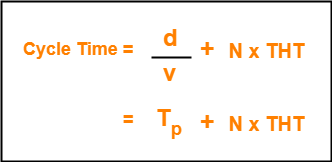Now, we start discussing about Token Passing Access Control Method.

## Token Passing-

In this access control method,

• All the stations are logically connected to each other in the form of a ring.
• The access of stations to the transmission link is governed by a token.
• A station is allowed to transmit a data packet if and only if it possess the token otherwise not.
• Each station passes the token to its neighboring station either clockwise or anti-clockwise.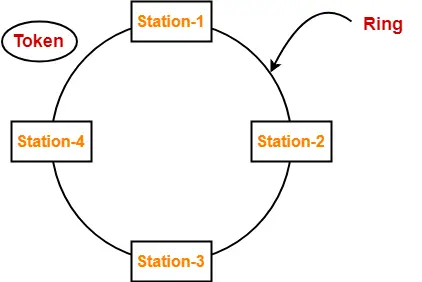## Assumptions-

Token passing method assumes-

• Each station in the ring has the data to send.
• Each station sends exactly one data packet after acquiring the token.

## Efficiency-

 Efficiency (η) = Useful Time / Total Time

In one cycle,

• Useful time = Sum of transmission delay of N stations since each station sends 1 data packet = N x Tt
• Total Time = Cycle time = Tp + N x THT

Thus,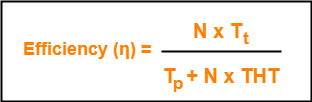Token Holding Time depends on the strategy implemented.

## Token Passing Strategies-

The following 2 strategies are used in token passing-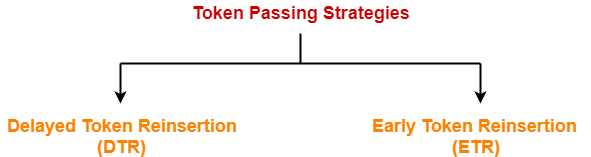1. Delayed Token Reinsertion (DTR)
2. Early Token Reinsertion (ETR)

## 1. Delayed Token Reinsertion-

In this strategy,

• Station keeps holding the token until the last bit of the data packet transmitted by it takes the complete revolution of the ring and comes back to it.

## Working-

After a station acquires the token,

• It transmits its data packet.
• It holds the token until the data packet reaches back to it.
• After data packet reaches to it, it discards its data packet as its journey is completed.
• It releases the token.

The following diagram illustrates these steps for station-1. Same procedure is repeated at every station.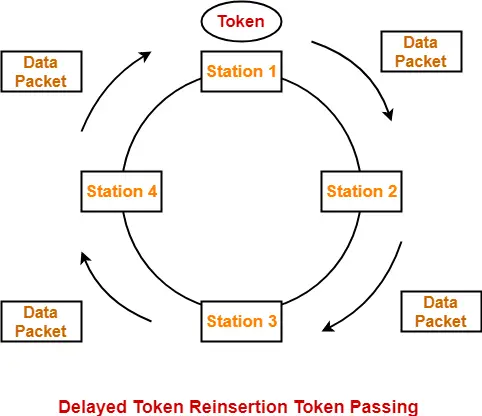## Token Holding Time-

 Token Holding Time (THT) = Transmission delay + Ring Latency

We know,

• Ring Latency = Tp + N x bit delay
• Assuming bit delay = 0 (in most cases), we get-

 Token Holding Time = Tt + Tp

## Efficiency-

Substituting THT = Tt + Tp in the efficiency expression, we get-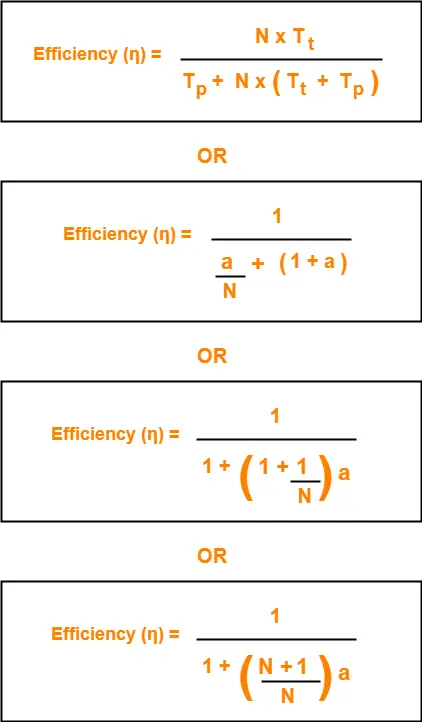## 2. Early Token Reinsertion-

In this strategy,

• Station releases the token immediately after putting its data packet to be transmitted on the ring.

## Working-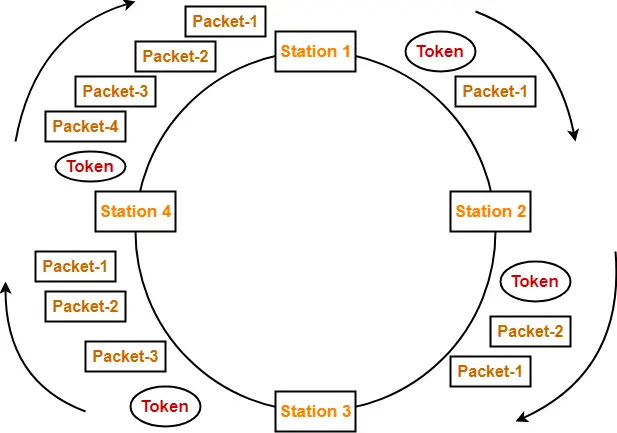### Step-01: At Station-1:

Station-1

• Acquires the token
• Transmits packet-1
• Releases the token

### Step-02: At Station-2:

Station-2

• Transmits packet-1
• Acquires the token
• Transmits packet-2
• Releases the token

### Step-03: At Station-3:

Station-3

• Transmits packet-1
• Transmits packet-2
• Acquires the token
• Transmits packet-3
• Releases the token

### Step-04: At Station-4:

Station-4

• Transmits packet-1
• Transmits packet-2
• Transmits packet-3
• Acquires the token
• Transmits packet-4
• Releases the token

### Step-05: At Station-1:

• Discards packet-1 (as its journey is completed)
• Transmits packet-2
• Transmits packet-3
• Transmits packet-4
• Acquires the token
• Transmits packet-1 (new)
• Releases the token

In this manner, the cycle continues.

## Token Holding Time-

 Token Holding Time (THT) = Transmission delay of data packet = Tt

## Efficiency-

Substituting THT = Tt in the efficiency expression, we get-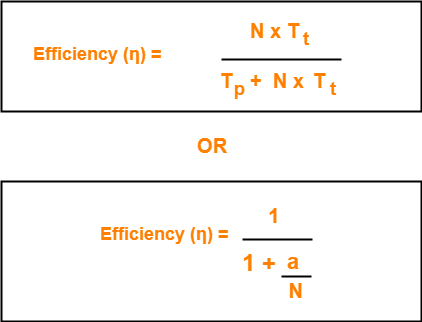## Differences between DTR and ETR-

 Delay Token Retransmission (DTR) Early Token Retransmission (ETR) Each station holds the token until its data packet reaches back to it. Each station releases the token immediately after putting its data packet on the ring. There exists only one data packet on the ring at any given instance. There exists more than one data packet on the ring at any given instance. It is more reliable than ETR. It is less reliable than DTR. It has low efficiency as compared to ETR. It has high efficiency as compared to ETR.

## Note-01:

In token passing,

• It is the responsibility of each transmitting station to remove its own data packet from the ring.

## Note-02:

While solving questions,

• If the strategy used is not mentioned, then consider Early Token Retransmission strategy.

To gain better understanding about Token Passing Method,

Watch this Video Lecture

Next Article- Practice Problems On Token Passing

Get more notes and other study material of Computer Networks.

Watch video lectures by visiting our YouTube channel LearnVidFun.

## CSMA / CD Protocol-

Before you go through this article, make sure that you have gone through the previous article on CSMA / CD Protocol.

We have discussed-

• CSMA / CD stands for Carrier Sense Multiple Access / Collision Detection.
• It allows the stations to sense the carrier and transmit data if the carrier is free.

The following CSMA / CD flowchart shows the CSMA / CD procedure-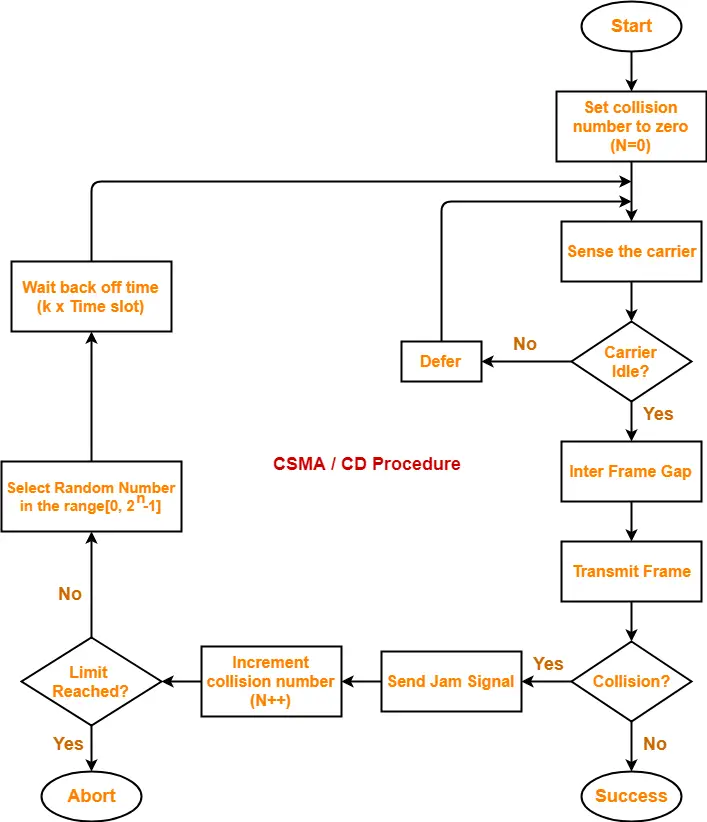## Back Off Time-

In CSMA / CD protocol,

• After the occurrence of collision, station waits for some random back off time and then retransmits.
• This waiting time for which the station waits before retransmitting the data is called as back off time.
• Back Off Algorithm is used for calculating the back off time.

## Back Off Algorithm-

After undergoing the collision,

• Transmitting station chooses a random number in the range [0, 2n-1] if the packet is undergoing collision for the nth time.
• If station chooses a number k, then-

 Back off time = k x Time slot

where value of one time slot = 1 RTT

## Example-

Consider the following scenario where stations A and D start transmitting their data simultaneously-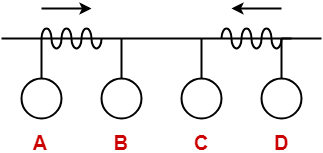For simplicity,

• We consider the value of time slot = 1 unit.
• Thus, back off time = K units.

## Scene-01: For 1st Data Packet Of Both Stations-

• Both the stations start transmitting their 1st data packet simultaneously.
• This leads to a collision.
• Clearly, the collision on both the packets is occurring for the 1st time.
• So, collision number for the 1st data packet of both the stations = 1.

## At Station A-

After detecting the collision,

• Station A randomly chooses a number in the range [0, 21-1] = [0,1].
• If station A chooses the number KA, then back off time = KA units.

## At Station D-

After detecting the collision,

• Station D randomly chooses a number in the range [0, 21-1] = [0,1].
• If station D chooses the number KD, then back off time = KD units.

Following 4 cases are possible-

 KA KD Remarks 0 0 In this case, both the stations start retransmitting their data immediately.This case leads to a collision again. 0 1 In this case, station A starts retransmitting its data immediately while station D waits for 1 unit of time.This case leads to A successfully retransmitting its data after the 1st collision. 1 0 In this case, station A waits for 1 unit of time while station D starts retransmitting its data immediately.This case leads to D successfully retransmitting its data after the 1st collision. 1 1 In this case, both the stations wait for 1 unit of time and then starts retransmitting their data simultaneously.This case leads to a collision again.

From here,

• Probability of station A to successfully retransmit its data after the 1st collision = 1 / 4
• Probability of station D to successfully retransmit its data after the 1st collision = 1 / 4
• Probability of occurrence of collision again after the 1st collision = 2 / 4 = 1 / 2

Now,

• Consider case-02 occurs.
• This causes station A to successfully retransmit its 1st packet after the 1st collision.

## Scene-02: For 2nd Data Packet Of Station A And 1st Data Packet Of Station D-

Consider after some time,

• Station A starts transmitting its 2nd data packet and station D starts retransmitting its 1st data packet simultaneously.
• This leads to a collision.

## At Station A-

• The 2nd data packet of station A undergoes collision for the 1st time.
• So, collision number for the 2nd data packet of station A = 1.
• Now, station A randomly chooses a number in the range [0, 21-1] = [0,1].
• If station A chooses the number KA, then back off time = KA units.

## At Station D-

• The 1st data packet of station D undergoes collision for the 2nd time.
• So, collision number for the 1st data packet of station D = 2.
• Now, station D randomly chooses a number in the range [0, 22-1] = [0,3].
• If station D chooses the number KD, then back off time = KD units.

Following 8 cases are possible-

 KA KD Remarks 0 0 In this case, both the stations start retransmitting their data immediately.This case leads to a collision again. 0 1 In this case, station A starts retransmitting its data immediately while station D waits for 1 unit of time.This case leads to A successfully retransmitting its data after the 2nd collision. 0 2 In this case, station A starts retransmitting its data immediately while station D waits for 2 unit of time.This case leads to A successfully retransmitting its data after the 2nd collision. 0 3 In this case, station A starts retransmitting its data immediately while station D waits for 3 unit of time.This case leads to A successfully retransmitting its data after the 2nd collision. 1 0 In this case, station A waits for 1 unit of time while station D starts retransmitting its data immediately.This case leads to D successfully retransmitting its data after the 2nd collision. 1 1 In this case, both the stations wait for 1 unit of time and then starts retransmitting their data simultaneously.This case leads to a collision again. 1 2 In this case, station A waits for 1 unit of time while station D waits for 2 unit of time.This case leads to A successfully retransmitting its data after the 2nd collision. 1 3 In this case, station A waits for 1 unit of time while station D waits for 3 unit of time.This case leads to A successfully retransmitting its data after the 2nd collision.

From here,

• Probability of station A to successfully retransmit its data after the 2nd collision = 5 / 8
• Probability of station D to successfully retransmit its data after the 2nd collision = 1 / 8
• Probability of occurrence of collision again after the 2nd collision = 2 / 8 = 1 / 4

Now,

• Consider case-03 occurs.
• This causes station A to successfully retransmit its 2nd packet after the 2nd collision.

## Scene-03: For 3rd Data Packet Of Station A And 1st Data Packet Of Station D-

Consider after some time,

• Station A starts transmitting its 3rd data packet and station D starts retransmitting its 1st data packet simultaneously.
• This leads to a collision.

## At Station A-

• The 3rd data packet of station A undergoes collision for the 1st time.
• So, collision number for the 3rd data packet of station A = 1.
• Now, station A randomly chooses a number in the range [0, 21-1] = [0,1].
• If station A chooses the number KA, then back off time = KA unit.

## At Station D-

• The 1st data packet of station D undergoes collision for the 3rd time.
• So, collision number for the 1st data packet of station D = 3.
• Now, station D randomly chooses a number in the range [0, 23-1] = [0,7].
• If station D chooses the number KD, then back off time = KD unit.

Following 16 cases are possible-

From here,

• Probability of station A to successfully retransmit its data after the 3rd collision = 13 / 16
• Probability of station D to successfully retransmit its data after the 3rd collision = 1 / 16
• Probability of occurrence of collision again after the 3rd collision = 1 / 16

In the similar manner, the procedure continues.

## Note-01:

With each successive collision-

• Back off time increases exponentially.
• Collision probability decreases exponentially.

## Note-02:

Back Off Algorithm is also known as Binary Exponential Back Off Algorithm because-

• It works for only two stations.
• The back off time increases exponentially.
• Collision probability decreases exponentially.

## Note-03:

• One disadvantage of Back Off Algorithm is that it shows capture effect.
• It means if a particular station wins the collision one time, then its probability of winning the successive collisions increases exponentially.

To gain better understanding about Back Off Algorithm,

Watch this Video Lecture

Next Article- Practice Problems On CSMA / CD & Back Off Algorithm

Get more notes and other study material of Computer Networks.

Watch video lectures by visiting our YouTube channel LearnVidFun.

## Access Control in Networking-

Before you go through this article, make sure that you have gone through the previous article on Access Control.

We have discussed-

• Access Control is a mechanism that controls the access of stations to the transmission link.
• There are various access control methods-## CSMA / CD-

 CSMA / CD stands for Carrier Sense Multiple Access / Collision Detection.

This access control method works as follows-

## Step-01: Sensing the Carrier-

• Any station willing to transmit the data senses the carrier.
• If it finds the carrier free, it starts transmitting its data packet otherwise not.

## How?

• Each station can sense the carrier only at its point of contact with the carrier.
• It is not possible for any station to sense the entire carrier.
• Thus, there is a huge possibility that a station might sense the carrier free even when it is actually not.

## Example-

Consider the following scenario-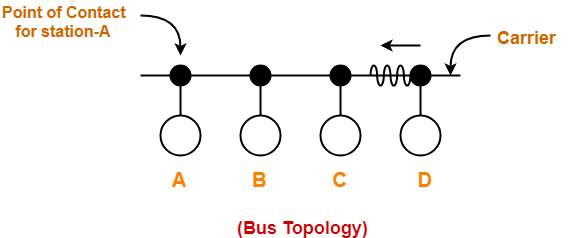At the current instance,

• If station A senses the carrier at its point of contact, then it will find the carrier free.
• But the carrier is actually not free because station D is already transmitting its data.
• If station A starts transmitting its data now, then it might lead to a collision with the data transmitted by station D.

## Step-02: Detecting the Collision-

In CSMA / CD,

• It is the responsibility of the transmitting station to detect the collision.
• For detecting the collision, CSMA / CD implements the following condition.
• This condition is followed by each station-

 Transmission delay >= 2 x Propagation delay

## Meaning-

According to this condition,

• Each station must transmit the data packet of size whose transmission delay is at least twice its propagation delay.
• If the size of data packet is smaller, then collision detection would not be possible.

## Length Of Data Packet-

We know-

• Transmission delay = Length of data packet (L) / Bandwidth (B)
• Propagation delay = Distance between the two stations (D) / Propagation speed (V)

Substituting values in the above condition, we get-

L / B >= 2 x D / V

Thus,

 L >= 2 x B x D / V

### Understanding the Condition To Detect Collision With Example

• Consider at time 10:00 am, station A senses the carrier.
• It finds the carrier free and starts transmitting its data packet to station D.
• Let the propagation delay be 1 hour.

(We are considering station D for the worst case)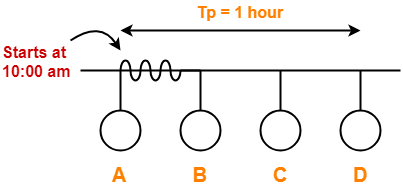• Let us consider the scenario at time 10:59:59:59 when the packet is about to reach the station D.
• At this time, station D senses the carrier.
• It finds the carrier free and starts transmitting its data packet.
• Now, as soon as station D starts transmitting its data packet, a collision occurs with the data packet of station A at time 11:00 am.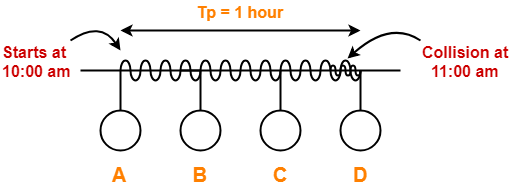• After collision occurs, the collided signal starts travelling in the backward direction.
• The collided signal takes 1 hour to reach the station A after the collision has occurred.
• For station A to detect the collided signal, it must be still transmitting the data.
• So, transmission delay of station A must be >= 1 hour + 1 hour >= 2 hours to detect the collision.
• That is why, for detecting the collision, condition is Tt >= 2Tp.

Two cases are possible-

### Case-01:

If no collided signal comes back during the transmission,

• It indicates that no collision has occurred.
• The data packet is transmitted successfully.

### Case-02:

If the collided signal comes back during the transmission,

• It indicates that the collision has occurred.
• The data packet is not transmitted successfully.
• Step-03 is followed.

## Step-03: Releasing Jam Signal-

• Jam signal is a 48 bit signal.
• It is released by the transmitting stations as soon as they detect a collision.
• It alerts the other stations not to transmit their data immediately after the collision.
• Otherwise, there is a possibility of collision again with the same data packet.
• Ethernet sends the jam signal at a frequency other than the frequency of data signals.
• This ensures that jam signal does not collide with the data signals undergone collision.

## Step-04: Waiting For Back Off Time-

• After the collision, the transmitting station waits for some random amount of time called as back off time.
• After back off time, it tries transmitting the data packet again.
• If again the collision occurs, then station again waits for some random back off time and then tries again.
• The station keeps trying until the back off time reaches its limit.
• After the limit is reached, station aborts the transmission.
• Back off time is calculated using Back Off Algorithm.

## CSMA / CD Flowchart-

The following CSMA / CD flowchart represents the CSMA / CD procedure-## Efficiency-

 Efficiency (η) = Useful Time / Total Time

Before a successful transmission,

• There may occur many number of collisions.
• 2 x Tp time is wasted during each collision.

Thus,

• Useful time = Transmission delay of data packet = Tt
• Useless time = Time wasted during collisions + Propagation delay of data packet = c x 2 x Tp + Tp
• Here, c = Number of contention slots / collision slots.

Thus,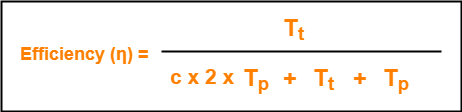Here,

• c is a variable.
• This is because number of collisions that might occur before a successful transmission are variable.

Probabilistic Analysis shows-

 Average number of collisions before a successful transmission = e

Substituting c = e in the above relation, we get-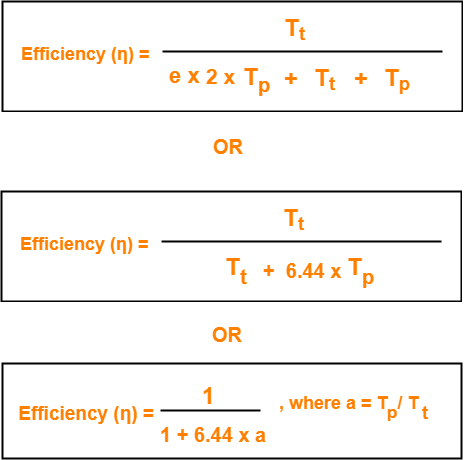## Probabilistic Analysis-

Let us perform the probabilistic analysis to find the average number of collisions before a successful transmission.

Consider-

• Number of stations connected to a CSMA / CD network = n
• Probability of each station to transmit the data = p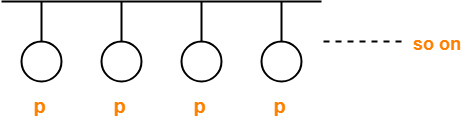Transmission will be successful only when-

• One station transmits the data
• Other (n-1) stations do not transmit the data.

Thus, Probability of successful transmission is given by-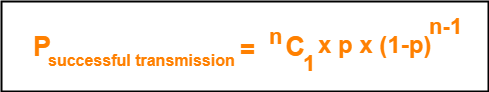Now, let us find the maximum value of Psuccessful transmission.

For maximum value, we put-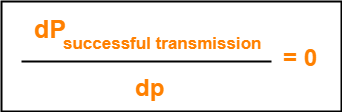On solving,

 At p = 1/n, we get the maximum value of Psuccessful transmission

Thus,

(Psuccessful transmission)max

= nC1 x 1/n x (1 – 1/n)n-1

= n x 1/n x (1 – 1/n)n-1

= (1 – 1/n)n-1

 (Psuccessful transmission)max = (1 – 1/n)n-1

If there are sufficiently large number of stations i.e. n → ∞, then we have-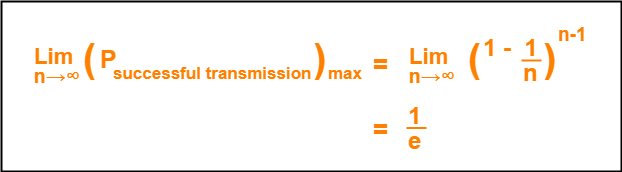Number of times a station must try before successfully transmitting the data packet

= 1 / Pmax         (Using Poisson’s distribution)

= 1 / (1/e)

= e

From here, we conclude-

Average number of collisions that might occur before a successful transmission = e

## Note-01:

• CSMA / CD is used in wired LANs.
• CSMA / CD is standardized in IEEE 802.3

## Note-02:

• CSMA / CD only minimizes the recovery time.
• It does not take any steps to prevent the collision until it has taken place.

## Important Formulas-

• Condition to detect collision: Transmission delay >= 2 x Propagation delay
• Minimum length of data packets in CSMA / CD = 2 x Bandwidth x Distance / Speed
• Efficiency of CSMA / CD = 1 / (1 + 6.44 x a) where a = Tp / Tt
• Probability of successful transmission = nC1 x p x (1-p)n-1
• Average number of collisions before a successful transmission = e

To gain better understanding about CSMA / CD,

Watch this Video Lecture

Next Article- Binary Exponential Back Off Algorithm

Get more notes and other study material of Computer Networks.

Watch video lectures by visiting our YouTube channel LearnVidFun.